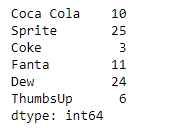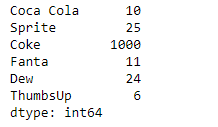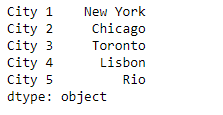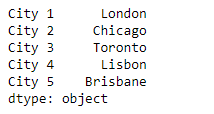# Python | Pandas Series.replace()

Pandas series is a One-dimensional ndarray with axis labels. The labels need not be unique but must be a hashable type. The object supports both integer- and label-based indexing and provides a host of methods for performing operations involving the index.

Pandas` Series.replace()` function is used to replace values given in to_replace with value. The values of the Series are replaced with other values dynamically.

Syntax: Series.replace(to_replace=None, value=None, inplace=False, limit=None, regex=False, method=’pad’)

Parameter :
to_replace : How to find the values that will be replaced.
value : Value to replace any values matching to_replace with.
inplace : If True, in place.
limit : Maximum size gap to forward or backward fill.
regex : Whether to interpret to_replace and/or value as regular expressions
method : The method to use when for replacement, when to_replace is a scalar, list or tuple and value is None.

Returns : Object after replacement.

Example #1: Use `Series.replace()` function to replace some values from the given Series object.

 `# importing pandas as pd ` `import` `pandas as pd ` ` `  `# Creating the Series ` `sr ``=` `pd.Series([``10``, ``25``, ``3``, ``11``, ``24``, ``6``]) ` ` `  `# Create the Index ` `index_ ``=` `[``'Coca Cola'``, ``'Sprite'``, ``'Coke'``, ``'Fanta'``, ``'Dew'``, ``'ThumbsUp'``] ` ` `  `# set the index ` `sr.index ``=` `index_ ` ` `  `# Print the series ` `print``(sr) `

Output :Now we will use `Series.replace()` function to replace the old values with the new ones.

 `# replace 3 by 1000 ` `result ``=` `sr.replace(to_replace ``=` `3``, value ``=` `1000``) ` ` `  `# Print the result ` `print``(result) `

Output :As we can see in the output, the `Series.replace()` function has successfully replaced the old value with the new one.

Example #2 : Use `Series.replace()` function to replace some values from the given Series object.

 `# importing pandas as pd ` `import` `pandas as pd ` ` `  `# Creating the Series ` `sr ``=` `pd.Series([``'New York'``, ``'Chicago'``, ``'Toronto'``, ``'Lisbon'``, ``'Rio'``]) ` ` `  `# Create the Index ` `index_ ``=` `[``'City 1'``, ``'City 2'``, ``'City 3'``, ``'City 4'``, ``'City 5'``]  ` ` `  `# set the index ` `sr.index ``=` `index_ ` ` `  `# Print the series ` `print``(sr) `

Output :Now we will use `Series.replace()` function to replace the old values with the new ones using a list.

 `# replace the old ones in the list with  ` `# the new values ` `result ``=` `sr.replace(to_replace ``=` `[``'New York'``, ``'Rio'``], value ``=` `[``'London'``, ``'Brisbane'``]) ` ` `  `# Print the result ` `print``(result) `

Output :As we can see in the output, the `Series.replace()` function has successfully replaced the old value with the new one using the list.

My Personal Notes arrow_drop_upCheck out this Author's contributed articles.

If you like GeeksforGeeks and would like to contribute, you can also write an article using contribute.geeksforgeeks.org or mail your article to contribute@geeksforgeeks.org. See your article appearing on the GeeksforGeeks main page and help other Geeks.

Please Improve this article if you find anything incorrect by clicking on the "Improve Article" button below.

Article Tags :

Be the First to upvote.

Please write to us at contribute@geeksforgeeks.org to report any issue with the above content.